# Brief notes on magnetic effects of electric current class 10

## Introduction

• From chapter electricity we know a little bit about electric field $\vec{E}$ and how it is produced at all points around it.
• Likewise magnets produces magnetic field at all points round it.
• We already learned about heating effects of electric current in previous chapter about electricity. Now in this chapter we shall study about another phenomenon related to current that is magnetic effects of current.
An electric current carrying wire behaves like a magnet:-
So, we already know that an electric current-carrying wire behaves like a magnet. Let us now describe an experiment that shows the presence of magnetic field near a current carrying wire.
ACTIVITY showing presence of magnetic field near current carrying wire:-
• This experiment is also called Oersted Experiment.
• First take a straight thick copper wire and place it between the points X and Y in an electric circuit, as shown in Fig. 1.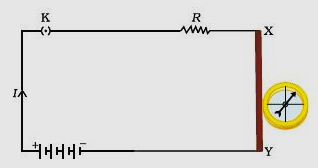Figure 1: compass needle is deflected when it is placed near a current carrying wire
• Now we place a small compass near to this copper wire.
• After placing the needle note the position of its needle.
• Now insert the key into the plug to close the circuit and pass the current through the circuit.
• Since the current is flowing in the circuit now observe the change in the position of the compass needle.
In the above activity we observed that the needle of the compass gets deflected when it is placed near the current carrying conductor. The result of this activity implies that current flowing through copper wire is producing a magnetic effect.
Thus we can say that electricity and magnetism are linked to each other.
Who was Hans Christian Oersted?
Hans Christian Oersted (1777-1851) through his experiments showed that electricity and magnetism are related to each other. His research later used in radio, television etc. The unit of magnetic field strength is name Oersted in his honour.

## Magnets and magnetism

• Magnets have been source of curiosity for ages. You can find magnets in places like laboratories, in toys, magnetic stickers that stick in refrigerator etc.
• So, what is a magnet? The substances which have the property of attracting small pieces of iron, nickel and cobalt etc. are called magnets and this property of attraction is called magnetism.
• Most of the metals like copper, gold, silver aluminium etc. are not attracted to magnets.
• Magnets are found naturally in certain natural rocks and can also be made artificially by certain methods.
• Artificially made permanent magnets are made in various shapes like bar, rod, disk, ring etc.
• In a bar, rod and horse shoe magnets north and south poles are dictated by letters N and S or North Pole is indicated by a dot.
• In disk and ring magnets one face is North Pole and another face is South Pole.
• Permanent magnets are commonly used as a direction finding compass.
What are magnetic poles?
agnetic poles refer to the two areas of a magnet where the magnetic effects are the strongest. The poles are generally termed as the north and south poles.

### Properties of magnet:

• Attracts objects of iron, cobalt and nickel.
• Force of attraction of a magnet is greater at its poles then in the middle.
• Like poles of magnets repel each other while unlike poles of magnets attract each other.
• A free suspended magnet always point towards north and south direction.
• The pole of a magnet which points toward north direction is called North Pole or north seeking.
• The pole of a magnet which points toward south direction is called South Pole or south seeking.

## Magnetic Field

• When we bring two magnets near each either they attract each other or they repel each other.
• We can explain this force of attraction and repulsion between two magnets using the concept of Magnetic Field.
• Magnets produces magnetic field in the space around it, which exerts force on any other magnet placed in this region. So it is the region around magnet within which its influence can be experienced.
What is magnetic Field?
The space around a magnet in which the force of attraction and repulsion due to the magnet can be detected is called the magnetic field.
IMPORTANT NOTE:- Each point in the field of any magnet has a particular strength and magnetic field at each point has definite direction.
How do you find the direction of the magnetic field due to magnet at a point near it?
The direction of the magnetic field due to a magnet at a point near it can be found by placing a magnetic compass at that point.  The compass needle gets deflected when it is placed near the magnet.
More about compass
• The simplest compass is a magnetized metal needle mounted in such a way that it can spin freely.
• Needle of a compass is a small bar magnet. This is the reason it gets deflected when we place it in the field of other magnet.
• Compass needles are lightweight because earth has very week magnetic field. To show up the effect of that tiny magnetic field it should have even less effect due to gravity (note:- that gravitational force is much stronger then the force produced by earth’s magnetic field).
• Compass needles are mounted on frictionless bearings because in this case there would be less frictional resistance for the magnetic force to overcome.
• The ends of a compass needle points approximately in North and south directions.

### About earth’s magnetism

• The earth has a magnetic field which we call as the earth’s magnetic field.
• The magnetic field is tilted slightly from the Earth’s axis.
• The core of earth is filled with molten iron (Fe) which give Earth its very own magnetic field.
• This large magnetic field protects the Earth from space radiation and particles such as the solar wind.
• The region surrounding Earth where its magnetic field is located is termed as the Magnetosphere.
• Earth has a magnetic field that has a shape similar to that of a large bar magnet.
• To the north is the magnetic north pole, which is really the south pole of Earth’s bar magnet.  (It is because this pole attracts the north pole of the compass magnet)

## Magnetic Field Lines

• Magnetic field surrounding the magnet and the force it exerts are depicted using imaginary curved lines with arrow called magnetic field lines.

### Ways of obtaining magnetic field lines around a bar magnet

(A) Iron filings demonstration

Procedure:-

1. Fix a sheet of white paper on a drawing board using some adhesive material.
2. Place a bar magnet in the centre of it.
3. Sprinkle some iron filings uniformly around the bar magnet (Fig. 2). A salt-sprinkler may be used for this purpose.
4. Now tap the board gently.
5. Iron filings near the bar magnet align themselves along the field lines.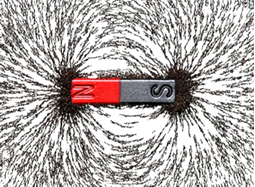Fig. 2 Iron filings near the bar magnet align themselves along the field lines. This happens because the magnet exerts its influence in the region surrounding it. Therefore the iron filings experience a force. The force thus exerted makes iron filings to arrange in a pattern. The lines along which the iron filings align themselves represent magnetic field lines.
(B) Demonstration using magnetic compass
With this demonstration you can draw the field lines of a bar magnet yourself

Procedure of this activity:-

1. Take a small compass and a bar magnet.
2. Place the magnet on a sheet of white paper fixed on a drawing board, using some adhesive material.
3. Mark the boundary of the magnet.
4. Place the compass near the north pole of the magnet.
5. Here you will notice that the south pole of the needle points towards the north pole of the magnet. The north pole of the compass is directed away from the north pole of the magnet.
6. Mark the position of two ends of the needle.
7. Now move the needle to a new position such that its south pole occupies the position previously occupied by its north pole.
8. In this way, proceed step by step till you reach the south pole of the magnet as shown in Fig. 3.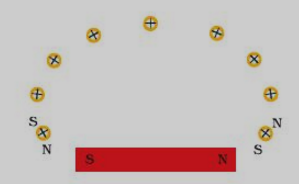Fig. 3 Drawing a magnetic field line with the help of a compass needle.
9. Join the points marked on the paper by a smooth curve. This curve represents a field line.
10. Repeat the above procedure and draw as many lines as you can. You will get a pattern shown in Fig. 4. These lines represent the magnetic field around the magnet. These are known as magnetic field lines.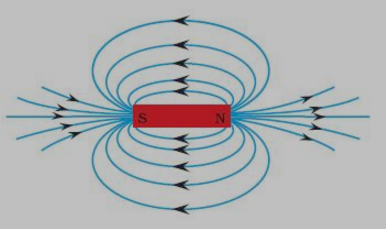Fig. 4 Field lines around a bar magnet
11. Observe the deflection in the compass needle as you move it along a field line. The deflection increases as the needle is moved towards the poles.

You can perform this activity in the link of simulation given below
https://cdac.olabs.edu.in/?sub=74&brch=9&sim=74&cnt=4

There is one other simulation link that you can use to visualise the field of the bar magnet.

How to run this simulation On clicking the 'click to run' button you will be asked to download a file(java file .jnlp). Once you download the file run the file and observe the magnetic field lines due to bar magnet. Please note that you need to have java installed in your system. For getting java you can visit this link

Observations

1. Direction in which compass needle points is the direction of the magnetic field.
2. The strength of the magnetic field is inversely proportional to the distance between the field lines.
3. Magnetic field lines never cross each other. It is unique at every point in space.
4. Magnetic field lines begin at the north pole of a magnet and terminate on the South Pole.

### Properties of magnetic field lines

1. All field lines are closed curves.
2. Outside the magnet field lines emerge from North Pole and merge at South Pole.
3. Inside a magnet, the direction of field lines is from South Pole to its north pole.
4. Field lines never intersect each other.
5. Field lines are closed together near the poles and spread out away from them.
6. The field is stronger where the field lines are more closely spaced. So, the field is stronger near the poles then at other points.
Why do field lines never intersect each other?
If two lines were to intersect each other, then a compass needle placed at the point of interaction would point in two different directions which is not possible.

## Magnetic Effect of Current

what is a magnetic effect of Current?
Electricity and Magnetism are related phenomenon. When an electric current is passed through metallic conductor, it generates a magnetic field around it.

Magnetic Field due to current through straight Conductor
Electric current through a straight Conductor generates magnetic field around it.
a)Magnetic Field intensity increases on the increasing the current in the conductor
b) Magnetic field decrease as the distance increase from the conductor
c) Magnetic Field direction can be find using Right Hand Thumb Rule

What is Right Hand Thumb rule?
When you are holding a current-carrying straight conductor in your right hand such that the thumb points towards the direction of current. Then your fingers will wrap around the conductor in the direction of the field lines of the magnetic field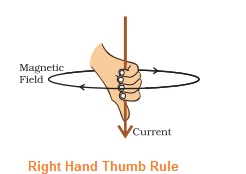## Magnetic Field due to a Current through a Circular Loop

a) As with straight conductor, the magnetic field lines would be in the form of concentric circles around every part of the periphery of the conductor.
b) The magnetic field would be stronger near the periphery of the loop as magnetic field lines tend to remain closer when near the conductor.
c) The magnetic field lines would be distant from each other when we move towards the centre of the current carrying loop. At the centre, the arcs of big circles would appear as straight lines

## Magnetic Field due to current through a coil having number of turns

We know that the magnetic field produced by a current-carrying wire at a given point depends directly on the current passing through it and the current in each circular turn has the same direction
Therefore, Magnitude of magnetic field gets summed up with increase in the number of turns of coil. If there are ‘n’ turns of coil, magnitude of magnetic field will be ‘n’ times of magnetic field in case of a single turn of coil.

## Solenoid

A coil of many circular turns of insulated copper wire wrapped closely in the shape of a cylinder is called a solenoid
Magnetic Effect of Current carrying Solenoid
A current carrying solenoid produces similar pattern of magnetic field as a bar magnet. One end of solenoid behaves as the north pole and another end behaves as the south pole. Magnetic field lines are parallel inside the solenoid; like a bar magnet; which shows that magnetic field is same at all points inside the solenoid
Electromagnet
When a piece of magnetic material, like soft iron is placed inside a solenoid,the  strong magnetic field produced inside a solenoid magnetise the soft iron and it behaves like strong magnet. The magnetism in the soft iron is temporary and it becomes null when the current is switched off. This type of magnet is called Electromagnets. So electromagnets are temporary magnets

## Force on a current carrying conductor in a Magnetic Field

When a current carrying, conductor is placed in a magnetic field, it experienced a force. The direction of force depends on the direction of the current and direction of the Magnetic Field. The direction of the force can be found using Fleming Left hand rule

Fleming Left hand rule
Stretch the thumb, forefinger and middle finger of your left hand such that they are mutually perpendicular. If the first finger points in the direction of magnetic field and the second finger in the direction of current, then the thumb will point in the direction of motion or the force acting on the conductor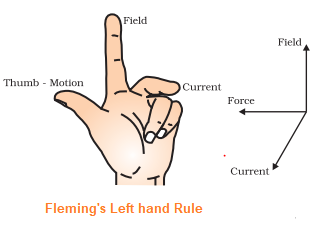Electric Motor
An electric motor is a device which works on the above principle. Here the electrical energy is converted to mechanical energy. Here a current carrying conductor is placed in the magnetic field and force acts on the conductor and it rotates and do the mechanical work.
This is used in electric fans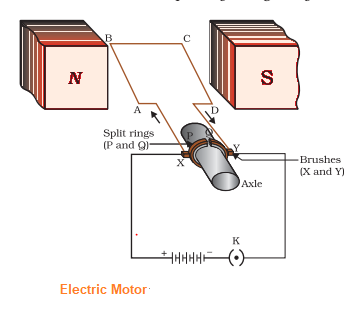Working of electric Motor
a. An electric motor, consists of a rectangular coil ABCD of insulated copper wire. The coil is placed between the two poles of a magnetic field such that the arm AB and CD are perpendicular to the direction of the magnetic field. The ends of the coil are connected to the two halves P and Q of a split ring.
b. When the electric current is switched on, Current in the coil ABCD enters through conducting brush X and flows back to the battery through brush Y. Current in arm AB of the coil flows from A to B. In arm CD it flows from C to D, that is, opposite to the direction of current through arm AB. On applying Fleming’s left hand rule for the direction of force on a current-carrying conductor in a magnetic field We find that the force acting on arm AB pushes it downwards while the force acting on arm CD pushes it upwards. Thus the coil and the axle O, mounted free to turn about an axis, rotate anti-clockwise.
c.At half rotation, Q makes contact with the brush X and P with brush Y. Therefore the current in the coil gets reversed and flows along the path DCBA. A device that reverses the direction of flow of current through a circuit is called a commutator. In electric motors, the split ring acts as a commutator. The reversal of current also reverses the direction of force acting on the two arms AB and CD. Thus the arm AB of the coil that was earlier pushed down is now pushed up and the arm CD previously pushed up is now pushed down. Therefore the coil and the axle rotate half a turn more in the same direction. The reversing of the current is repeated at each half rotation, giving rise to a continuous rotation of the coil and to the axle.

## Electromagnetic Induction

Electromagnetic induction is the production of induced current in a coil placed in a region where the magnetic field changes with time.
The magnetic field may change due
(a) relative motion between the coil and a magnet placed near to the coil.
(b) If the coil is placed near to a current-carrying conductor, the magnetic field may change either due to a change in the current through the conductor or due to the relative motion between the coil and conductor.
The direction of the induced current is given by the Fleming’s right-hand rule.

Fleming’s Right Hand rule
Stretch the thumb, forefinger and middle finger of right hand so that they are perpendicular to each other. If the forefinger indicates the direction of the magnetic field and the thumb shows the direction of motion of conductor, then the middle finger will show the direction of induced current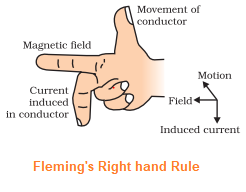Electric Generator
It is a device which converts mechanical energy into electrical energy. It is based on electromagnetic induction principle as explained above.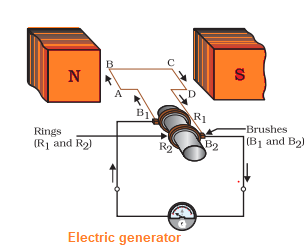Working of electric Generator
a. When the coil ABCD is rotated clockwise . By applying Fleming’s right-hand rule, the induced currents are set up in these arms along the directions AB and CD. Thus an induced current flows in the direction ABCD. This means that the current in the external circuit flows from B2 to B1.
b. After half a rotation, arm CD starts moving up and AB moving down. As a result, the directions of the induced currents in both the arms change, giving rise to the net induced current in the direction DCBA. The current in the external circuit now flows from B1 to B2. Thus after every half rotation the polarity of the current in the respective arms changes. Such a current, which changes direction after equal intervals of time, is called an alternating current (abbreviated as AC). This device is called an AC generator.
c. To get a direct current (DC, which does not change its direction with time), a split-ring type commutator must be used. With this arrangement, one brush is at all times in contact with the arm moving up in the field, while the other is in contact with the arm moving down. Thus a unidirectional current is produced. The generator is thus called a DC generator.

AC Current
which current direction changes after equal intervals of time, then the current is called an alternating current (abbreviated as AC).

Direct Current
When the current flows in the same direction and does not change direction, it is called Direct current (DC)

AC generator
Electric Generator can be used to generate both the AC and DC current. When it is designed to produce AC current, it is called AC generator

DC generator
Electric Generator can be used to generate both the AC and DC current. When it is designed to produce DC current, it is called DC generator

Facts about AC and DC currents
(a) Most power stations constructed these days produce AC.
(b) In India, the AC changes direction after every 1/100 second, that is, the frequency of AC is 50 Hz.
(c) An important advantage of AC over DC is that electric power can be transmitted over long distances without much loss of energy
Galvanometer
A galvanometer is an instrument that can detect the presence of a current in a circuit. The pointer remains at zero (the centre of the scale) for zero current flowing through it. It can deflect either to the left or to the right of the zero mark depending on the direction of current

## Domestic Electric Circuits

 S.no Terms Descriptions 1 Type of current/Potential Difference and Frequency AC electric power of 220 V with a frequency of 50 Hz. 2 Live Wire The wire, usually with red insulation cover, is called live wire (or positive) 3 Neutral Wire The wire, with black insulation, is called neutral wire (or negative). In our country, the potential difference between the Live wire and Neutral Wire is 220 V. 4 Earth Wire It has green insulation and this is connected to a metallic body deep inside earth. It is used as a safety measure to ensure that any leakage of current to a metallic body does not give any severe shock to a user. 5 Different Circuit used in the Home Supply Two separate circuits are used 1) 15 A current rating for appliances with higher power ratings such as geysers, air coolers, etc. 2) 5 A current rating for bulbs, fans, etc 6 Appliance circuit a) Each appliance has a separate switch to ‘ON’/‘OFF’ the flow of current through it. b) Each appliance has equal potential difference; they are connected parallel to each other 7 Electric Fuse a) A fuse in a circuit prevents damage to the appliances and the circuit due to overloading b) electric fuse prevents the electric circuit and the appliance from a possible damage by stopping the flow of unduly high electric current. c) Fuse is based on The Joule heating that takes place in the fuse melts it to break the electric circuit d) Tin-lead alloy is general used to make fuse wire

link to this page by copying the following text

### Practice Question

Question 1 Which among the following is not a base?
A) NaOH
B) $NH_4OH$
C) $C_2H_5OH$
D) KOH
Question 2 What is the minimum resistance which can be made using five resistors each of 1/2 Ohm?
A) 1/10 Ohm
B) 1/25 ohm
C) 10 ohm
D) 2 ohm
Question 3 Which of the following statement is incorrect? ?
A) For every hormone there is a gene
B) For production of every enzyme there is a gene
C) For every molecule of fat there is a gene
D) For every protein there is a gene